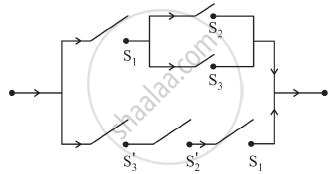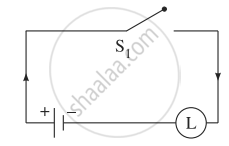# Give an alternative equivalent simple circuit for the following circuit: - Mathematics and Statistics

Sum

Give an alternative equivalent simple circuit for the following circuit:#### Solution

Let p: the switch S1 is closed
q: the switch S2 is closed
r: the switch S3 is closed
∼ q: the switch S2′ is closed or the switch S2 is open
∼ r: the switch S3′ is closed or the switch S3 is open.
Then the symbolic form of the given circuit is:
[p ∧ (q ∨ r)] ∨ (∼ r ∧ ∼ q ∧ p)
Using the laws of logic, we have
[p ∧ (q ∨ r)] ∨ (∼ r ∧ ∼ q ∧ p)
≡ [p ∧ (q ∨ r)] ∨ [∼ (r ∨ q) ∧ p] .......(By De Morgan’s Law)
≡ [p ∧ (q ∨ r)] ∨ [p ∧ ∼ (q ∨ r)] ......(By Commutative Law)
≡ p ∧ [(q ∨ r) ∨ ∼ (q ∨ r)] ...........(By Distributive Law)
≡ p ∧ T ...........(By Complement Law)
≡ p .........(By Identity Law)
Hence, the alternative equivalent simple circuit isConcept: Application of Logic to Switching Circuits
Is there an error in this question or solution?